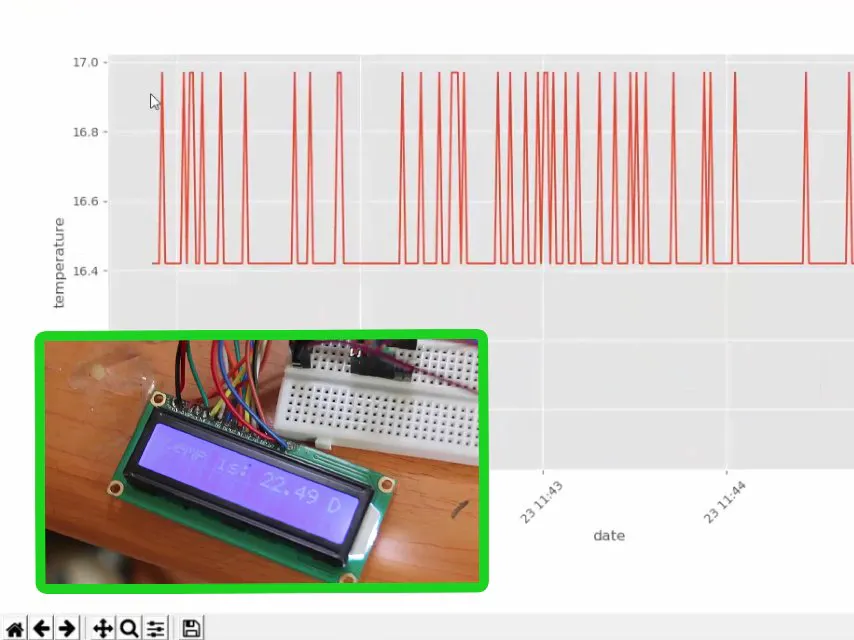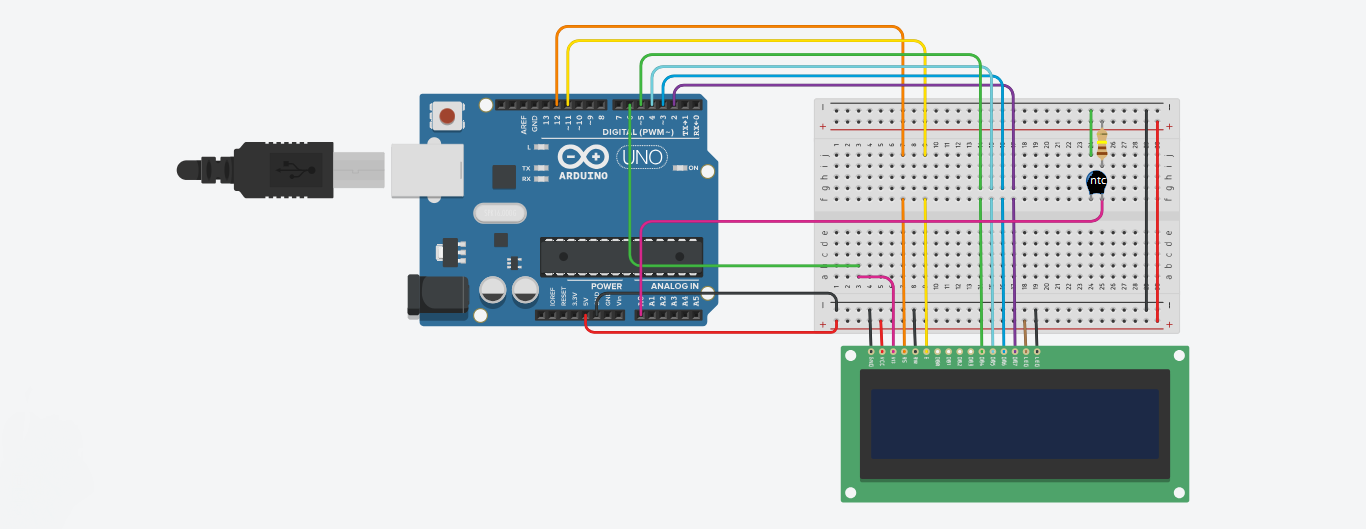Project tutorial# Arduino & Python3 Temperature Data Visualizer © GPL3+

This is an Arduino based project using an NTC thermistor to collect temperature data and Python 3 to save and visualize it.

• 6,846 views
• 29 respects

## Necessary tools and machines

 computer

## Apps and online services

This is a very simple project where we will use an ntc thermistor as a temperature captor an Arduino to get the value of resistance of the ntc thermistor and convert it to a valid data of temperature then send it to the computer using serial where we can keep and visualize it. Also we will use an lcd to display the temperature.

### Some details

The ntc thermistor is a variable resistor that change it value according to the temperature, ntc stands for negative temperature coefficient which means that the resistance drops with the rise of the temperature and from this given function:

R0 : is the values of the resistance when the temperature equal to T0

B : is a constant of the thermistor that you can find in the datasheet

We will find the expression of thetemperature by the resistance

The temperature here is in kelvins and we will use this function in the arduino code

Now let see how to measure the resistance of the thermistor using Arduino and we will use this circuit

Hooked to the 5v supply from the Arduino while R is a resistor of 110 ohm and the thermistor is Rt and we will measure the voltage Vt in the thermistor using analog input pin A0 and from the formula of voltage divider we will find the expression of the resistence Rt

### The circuit:

• LCD RS pin to digital pin 12
• LCD Enable pin to digital pin 11
• LCD D4 pin to digital pin 5
• LCD D5 pin to digital pin 4
• LCD D6 pin to digital pin 3
• LCD D7 pin to digital pin 2
• LCD R/W pin to ground
• LCD VSS pin to ground
• LCD VCC pin to 5V
• LCD K pin to ground
• LCD A pin to 5V
• LCD V0 pin to digital pin 6(pwm)
• LED to pin 13

Put a 110 ohm resistor in series with the thermistor, the side of 110 resitance to 5V, the side of the thermistor to GND and the point where the thermistor and the resistor meet to analog input A0:

## Code

##### arduino_serial_temperature.inoArduino
this is the arduino code
``` /*

The circuit:

* LCD RS pin to digital pin 12
* LCD Enable pin to digital pin 11
* LCD D4 pin to digital pin 5
* LCD D5 pin to digital pin 4
* LCD D6 pin to digital pin 3
* LCD D7 pin to digital pin 2
* LCD R/W pin to ground
* LCD VSS pin to ground
* LCD VCC pin to 5V
* LCD K pin to ground
* LCD A pin to 5V
* LCD V0 pin to digital pin 6
* LED to pin 13

put a 110 ohm resitance in series with the thermistor, the side of 110 resitance to 5V
the side of the thermistor to GND and the point where the thermistor and the resitence meet

*/

#include <LiquidCrystal.h>

LiquidCrystal lcd(12,11,5,4,3,2);//configure the lcd

double b;// an intermediate variable
double Vt;
double Rt;
int R=110;
int B=2800;
int R0=5;
int T0=25;
double T;
void setup() {
analogWrite(6,120);// configure the contrast of the lcd
lcd.begin(16,2);
Serial.begin(115200);// using serial to send data to the computer
}

void loop() {
b=analogRead(a);// get the value in the A0
Vt= (b/1024)*5;// convert it to voltage
Rt=(R*Vt)/(5-Vt);// measure the resistance of the thermistor
T=1/((log(Rt/R0)/B)+(1/(T0+273.15))); // get the temperature in kelvin
Serial.println(T-273.15);// send the temperature in using serial

// desplay the temperature in the lcd
lcd.clear();
lcd.print("temp is: ");
lcd.print(T-273.15);
lcd.print(" D");

// trigger the security alarm if the temperature is higher than 60 degree
if ((T-273.15)>60){
digitalWrite(13,HIGH);
delay(500);
digitalWrite(13,LOW);
delay(500);
}
else{
delay(1000);
}
// in the both cases there will be a delay of 1s between sending the temperature to the computer and display in in the lcd
}
```
##### live plot.pyPython
this is the python script for plotting the data you have to install mathplotlib library
```import matplotlib.pyplot as plt
import matplotlib.animation as animation
from matplotlib import style
import datetime as dta
'''configuring the plot'''
style.use('ggplot')
fig = plt.figure()

def animate(i):
dateconv = dta.datetime.fromtimestamp
'''define the function that gonna convert the unix time to date'''
'''read the data from the txt file'''
lines = graph_data.split('\n')
''' split the lines as a list'''
xs = []
''' x axis the tempurature'''
ys = []
''' y axis the date'''
for line in lines:
''' read the lines and append the data to ys ans xs'''
if len(line) > 1:
'''get only the non-empty line'''
x, y = line.split(',')
x=float(x)
y=float(y)
xs.append(x)
y = dateconv(y)
''' converte the unix time'''
ys.append(y)
'''plot the data'''
ax1.clear()
ax1.plot(ys, xs,label='temperature')
for label in ax1.xaxis.get_ticklabels():
'''set the rotation of the date into 45 degree in order to be readable'''
label.set_rotation(45)
plt.xlabel('date')
plt.ylabel('temperature')
plt.legend()
ani = animation.FuncAnimation(fig, animate, interval=1000)
'''animate the plot and refresh evry 1 second'''
plt.subplots_adjust(left=0.09, bottom=0.23, right=0.93, top=0.90, wspace=0.2, hspace=0)
'''just configurations'''
plt.show()
```
##### serial colector.pyPython
this is the python script to save the data to a txt file ,you have to install serial library
```from serial import Serial
import time
'''set the serial to connect to the arduino (you should change the port)'''
ser =Serial(
port='COM8',
baudrate = 115200,
timeout=None)
'''a function to add the data to the text file'''
date=time.time()
h=str(data)+','+str(date)+'\n'
fh = open('example.txt', 'a')
fh.write(h)
fh.close
while 1:
''' infinit loop'''
while(ser.inWaiting()==0):
'''wait for the data from serial'''
pass
'''add the data to the txt file'''
```

## Schematics#### Temperature and Humidity Data Logger

Project tutorial by Wimpie van den Berg

• 42,560 views
• 37 respects

#### SD Card Temperature Data Logger

Project tutorial by 3 developers

• 15,721 views
• 11 respects

#### Temperature and Humidity Data logger - Breadboard

Project tutorial by Jed Hodson

• 13,314 views
• 12 respects

#### Temperature Streaming with Arduino + Big Data Tools

Project showcase by Gabriel Rodriguez

• 17,259 views
• 39 respects

#### Arduino Temperature Control

Project tutorial by Team pandhoit

• 61,809 views